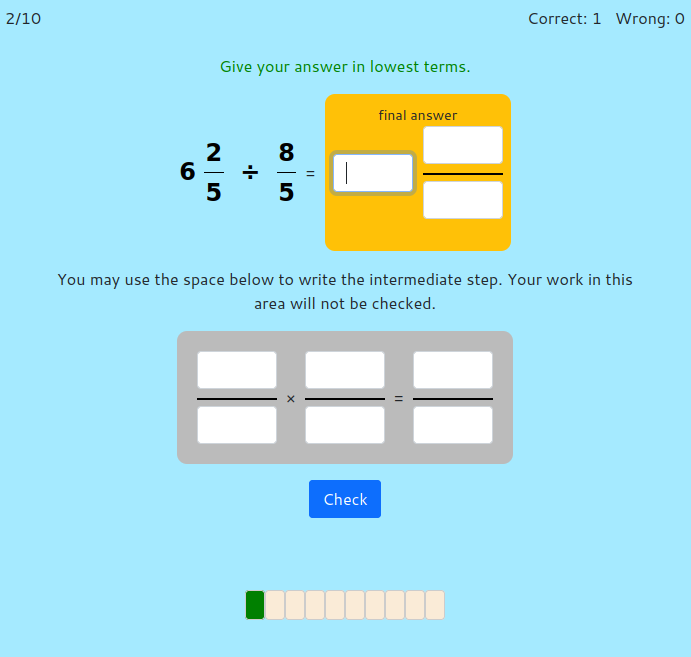MM Practice
×
Multiplication
Division
Place Value
Fractions & Decimals
Measurement
Statistics & Probability
Pre-Algebra
Money
Kindergarten

# Divide Fractions and Mixed NumbersOnline practice for grades 5-7

On this page, you can practice division of fractions and of mixed numbers. Each problem also includes a workspace where you can write the intermediate step: the corresponding fraction multiplication (since division of fractions is often solved by multiplying by a reciprocal). Your work in this additional workspace is not checked, only your final answer.

The options include one divided by unit fraction, unit fraction divided by a whole number, easy divisions done with mental math, fraction divided by a fraction, and mixed number divisions.

Note: ALL answers have to be given as mixed numbers, when possible. In other words, your answer cannot be left as an improper fraction.

Divide... (select at least one)

Screenshot:OR
Practice for a set time: min

Allow my comment to be posted on this site